Multiplying

In order to multiply two or more radical expressions of the same degree, the expressions under the radical sign can be multiplied.

Product Property of Roots: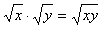Example 1 What is the product of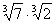?

Step 1. Verify the degree of the radicals are the same.

Both terms have a degree of 3.

Step 2. Use the Product Property of Roots to multiply the terms.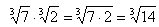Example 2 What is the product of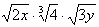? Assume all variables are positive.

Step 1. Verify the degree of the radicals are the same.

Two terms have a degree of two and can be multiplied, the term with degree 3 will stay the same.

Step 2. Use the Product Property of Roots to multiply the terms with the same degree.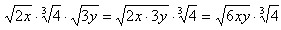Listen